# Non Verbal Reasoning - Classification

### Exercise :: Classification - Section 2

In each problem, out of the five figures marked (1), (2), (3), (4) and (5), four are similar in a certain manner. However, one figure is not like the other four. Choose the figure which is different from the rest.

31.

Choose the figure which is different from the rest.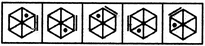(1)     (2)     (3)     (4)     (5)

 A. 1 B. 2 C. 3 D. 4 E. 5

Explanation:

In each one of the other figures, the small line segment lies one space ahead of the dot, in a CW direction.

32.

Choose the figure which is different from the rest.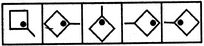(1)     (2)     (3)     (4)     (5)

 A. 1 B. 2 C. 3 D. 4 E. 5

Explanation:

In all other figures, the dot appears in the same corner of the square as the line outside it.

33.

Choose the figure which is different from the rest.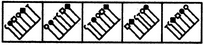(1)     (2)     (3)     (4)     (5)

 A. 1 B. 2 C. 3 D. 4 E. 5

Explanation:

In all other figures, the black rhombus appears adjacent to white square; the black circle appears adjacent to white circle and the clamp appears adjacent to the T-shaped element.

34.

Choose the figure which is different from the rest.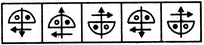(1)     (2)     (3)     (4)     (5)

 A. 1 B. 2 C. 3 D. 4 E. 5

Explanation:

In all other figures, both the arrowheads lie towards the diameter of the semicircle.

35.

Choose the figure which is different from the rest.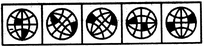(1)     (2)     (3)     (4)     (5)

 A. 1 B. 2 C. 3 D. 4 E. 5